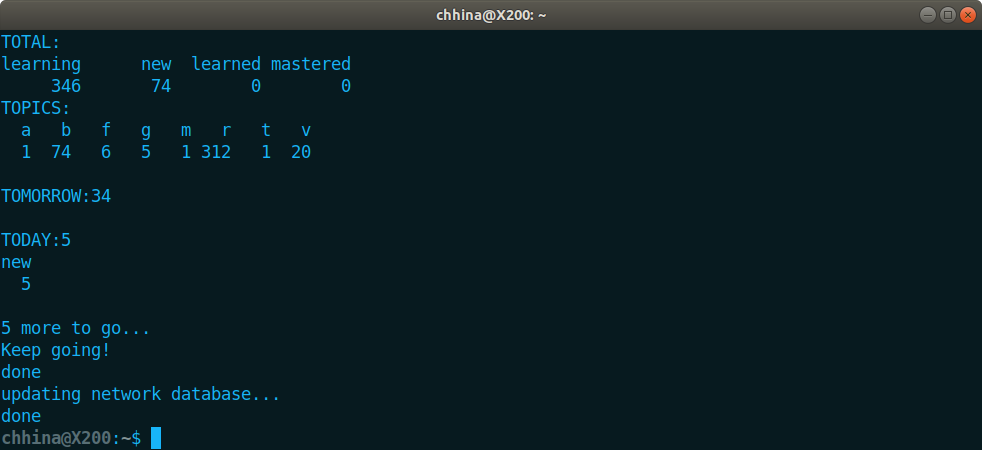# Quizme Workflow With Autoquiz

Friday, Dec 28, 2018
R

### Start autoquiz

You start the quiz by launching autoquiz at the terminal command prompt. Make sure the autoquiz script is in a place that is in your path environment. In my case I store my bash scripts in ~/bin directory.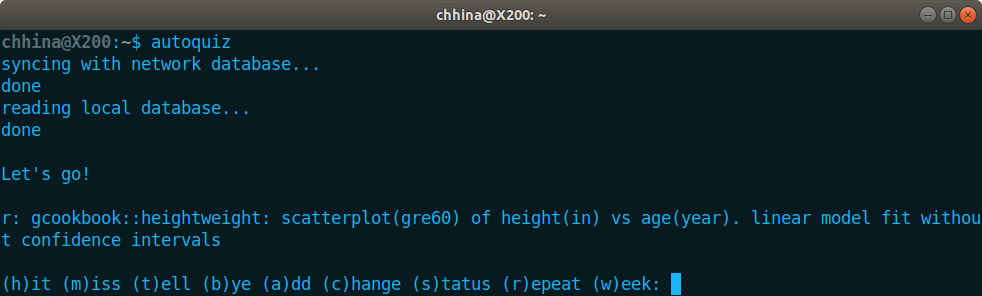Notice that first it updates the local quizme directory (~/.quizme) using the remote. Then it reads the local directory to load all objects in R environment. Then the first question is presented. The first letter (r:) in the question indicates that it's an R language question. We can try to solve the problem in a separate environment (R console or RStudio).

### Show the answer

To confirm if our answer is correct we can then use t command to see the solution: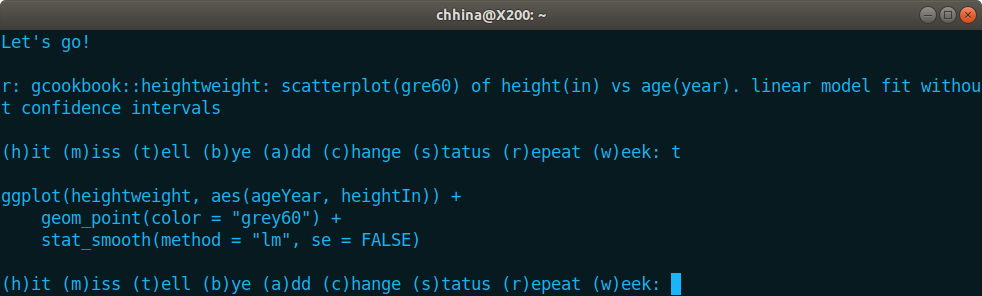### Edit a question

In the above problem, note that there was a typo in the question (grey60 was written as gre60). To edit the current question, we use c command: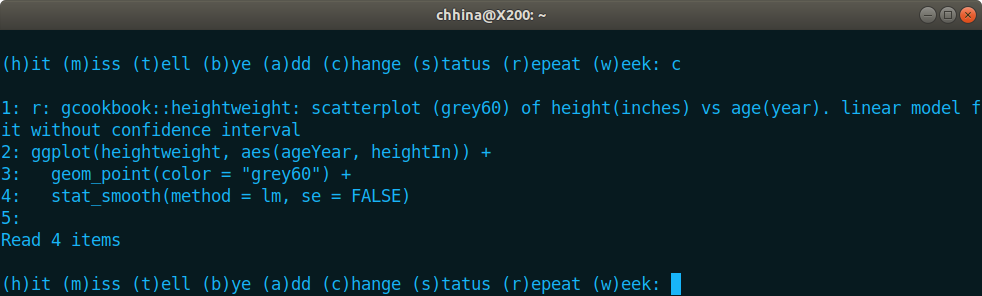It shows the line numbers now and allow you to add the question. Here we have to type the whole question again (it does not allow you to do a specific edit). I have not researched if there is a way to do that. Although not ideal, it gets the job done.

### Success Response

Assuming that our answer is correct, we use h to tell quizme that we successfully answered the question. Once we do that, it presents the next question. Most often in R, we are solving a problem related to some data. So I start my question with <package::dataset>. If the dataset is not a part of any package, I would store that in .quizme/data directory. In the following example, nyflights14.csv is stored in this directory.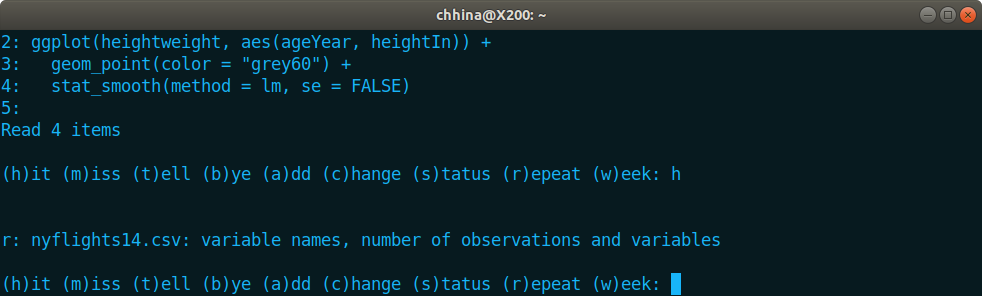### Fail Response

Let's say we do not recall the answer to this question. We will use m to tell quizme that we don't know the answer. Quizme poses us a new question and the missed question will be asked again soon.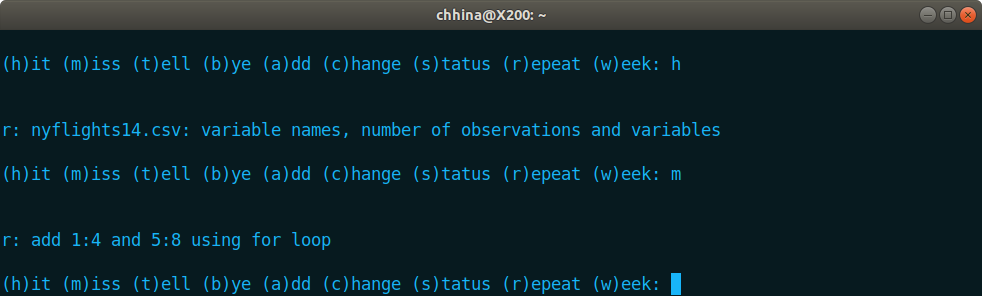### Adding a New Question

While thinking about the current question, let's assume we get distracted with some other topic that we don't know. We do a little bit of research and learn how to calculate probabilities of a normal distribution. It's good time to add our new knowledge into quizme. We do that using a command: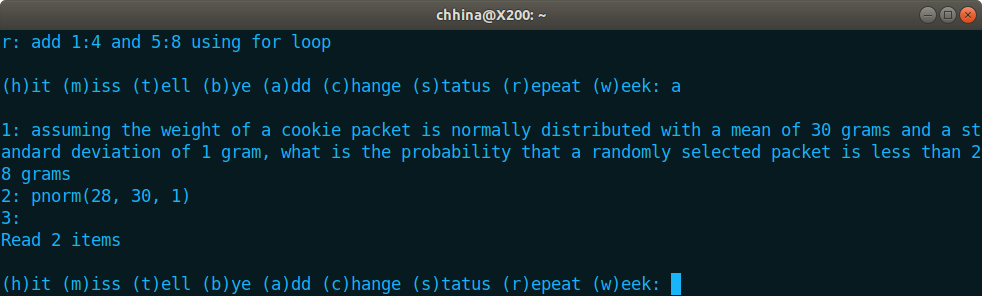Then we continue to solve our original problem. Using t, we ask quizme to show us the answer so we can confirm. Assuming that our answer was correct, we use h again. Note that the new question is the one which we missed earlier: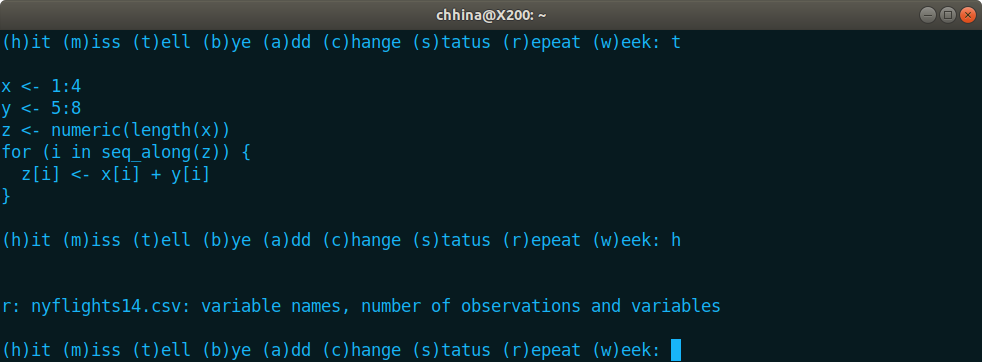### Checking Status

Ok, before we answer this question, we want to know where we are in the quiz (how many more questions to go and other stats). We will use s to show the status: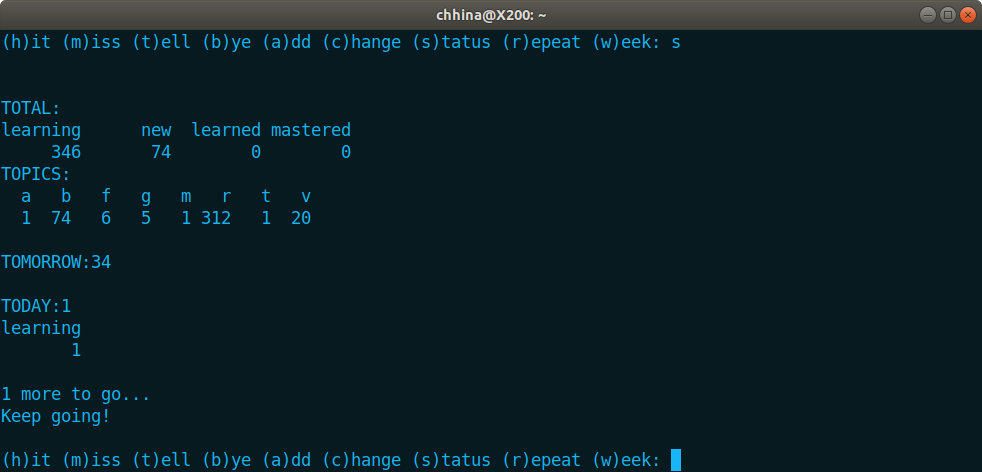First the total number of questions split by status and topics is shown. In this example, I am learning 346 questions and there are 74 new questions. I have not implemented criteria to move learning questions to learned and mastered yet. In addition, it shows how many questions are due tomorrow (34) and how many are remaining today (1, yippie!).

### Checking Weeks Workload

Besides looking at overall statistics, we can use w to see workload for the whole week. It shows number of questions for each day that are due in the next 7 days. This is helpful to plan your week.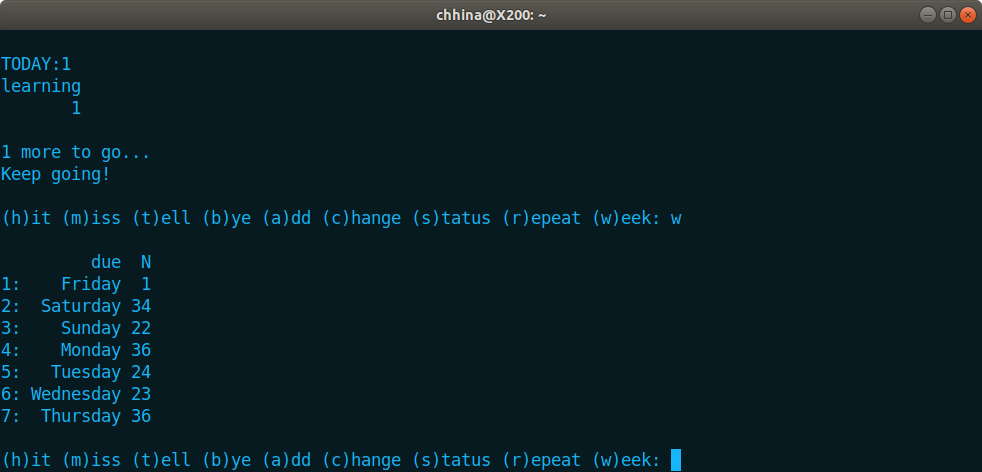### Display the last question

Alright, after looking at these stats, I'll typically forget the question that I was supposed to be working on. Instead of scrolling up the terminal, we can use r to repeat the last question: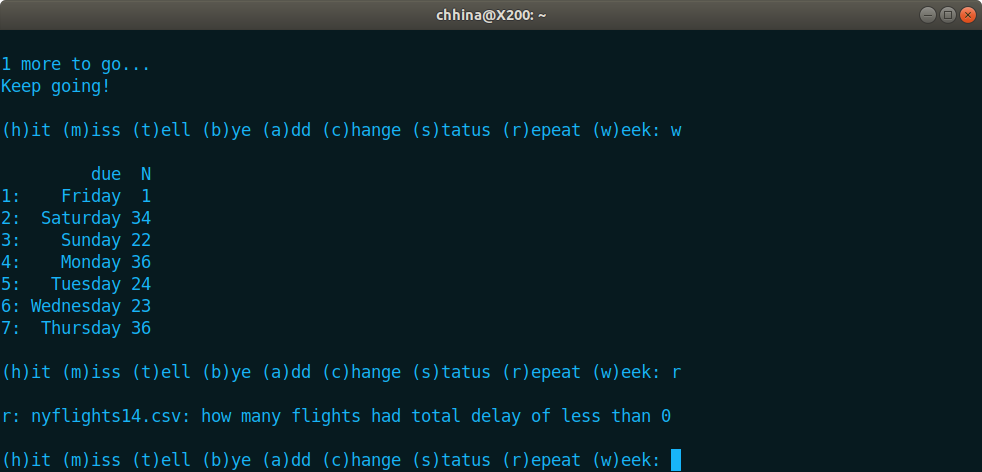### End of Quiz

Let's say we know the answer this time. We will use h to tell quizme just that. Since this was our last question, ‘Finished Quiz!!!’ message is displayed followed by overall stats. It detects that I did not learn any new questions today and automatically adds 5 new questions from the repository.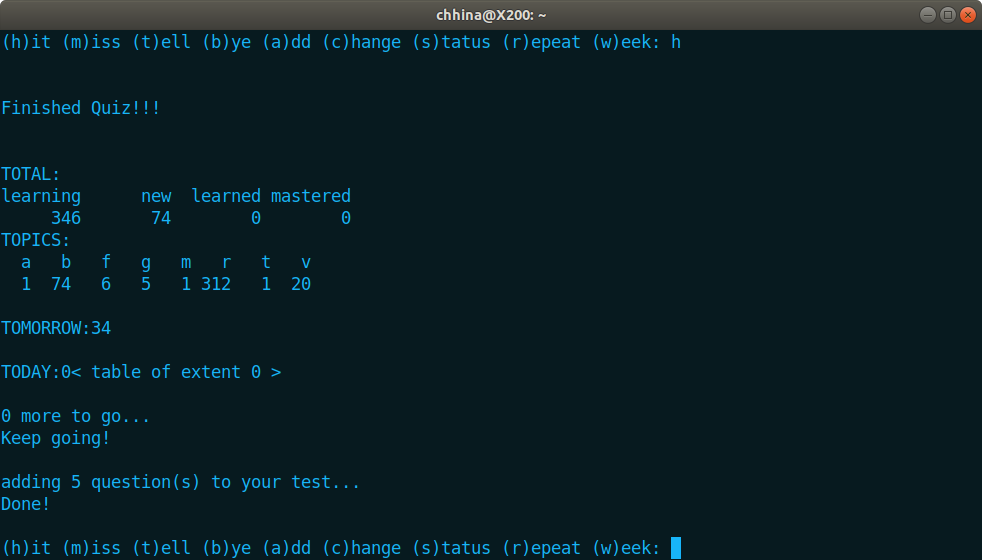### End of Session

Now we are really spent and plan to tackle the new questions sometime later, so we use b to call bye. It updates the local files first followed by updating the network files and we are done!